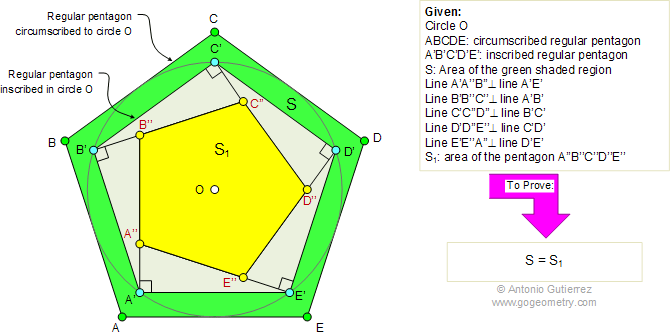Home Geometry Problems All Problems Visual Index 421-430 Solution / comment        By Antonio Gutierrez
 Problem 430: Circumscribed and Inscribed Regular Pentagon, Perpendicular, Areas. Level: High School, College, SAT Prep. In the figure, ABCDE is a regular pentagon circumscribed to circle O and A'B'C'D'E' is a regular pentagon inscribed in circle O. Lines A'A''B', B'B''C'', C'C''D'', D'D''E'', and E'E''A'' are perpendicular to A'E', A'B', B'C', C'D', and D'E', respectively. If S is the area of the green shaded region and S1 is the area of the pentagon A''B''C''D''E'', prove that S = S1.Recent Additions